# Advanced Data Structures Chapter 16 Advanced Data Structures

• Slides: 22
Download presentation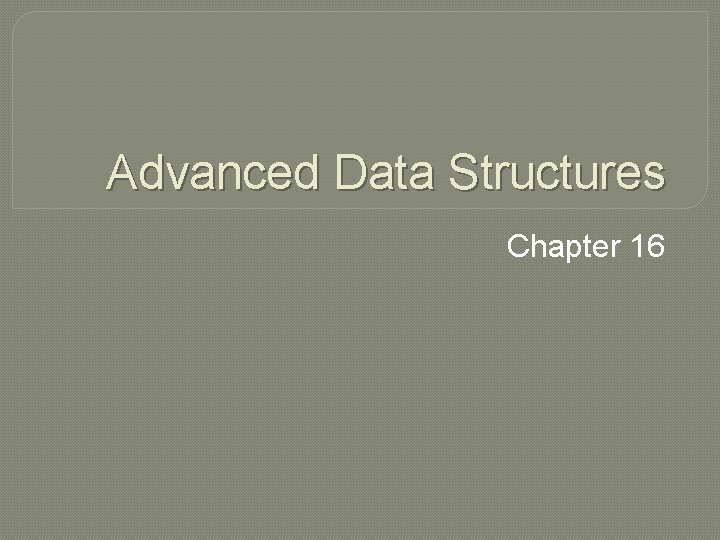Advanced Data Structures Chapter 16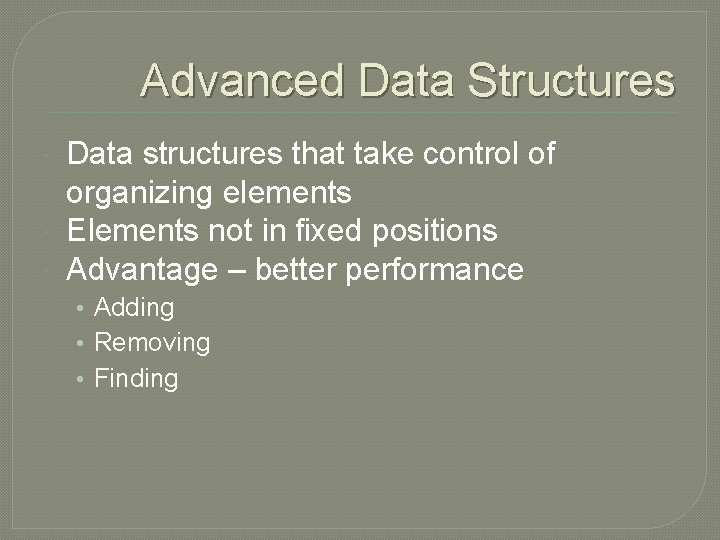Advanced Data Structures Data structures that take control of organizing elements Elements not in fixed positions Advantage – better performance • Adding • Removing • Finding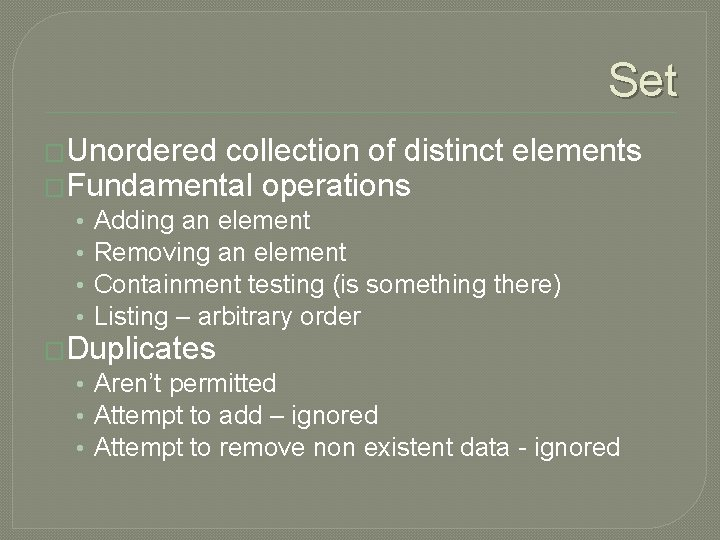Set �Unordered collection of distinct elements �Fundamental operations • • Adding an element Removing an element Containment testing (is something there) Listing – arbitrary order �Duplicates • Aren’t permitted • Attempt to add – ignored • Attempt to remove non existent data - ignored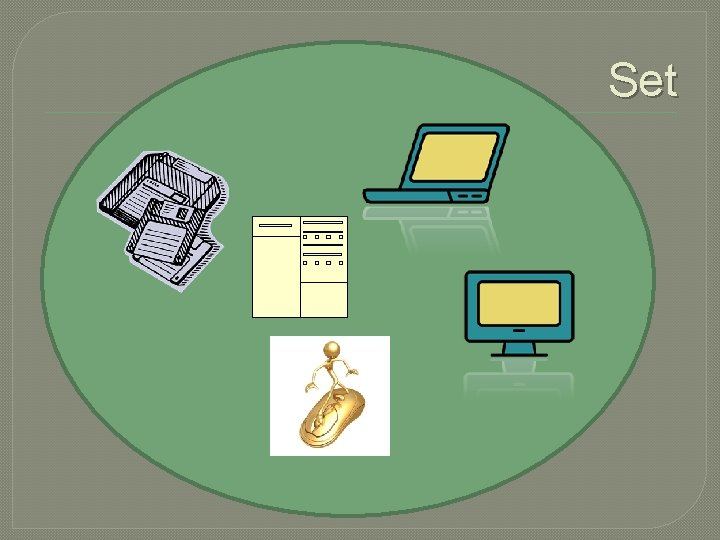Set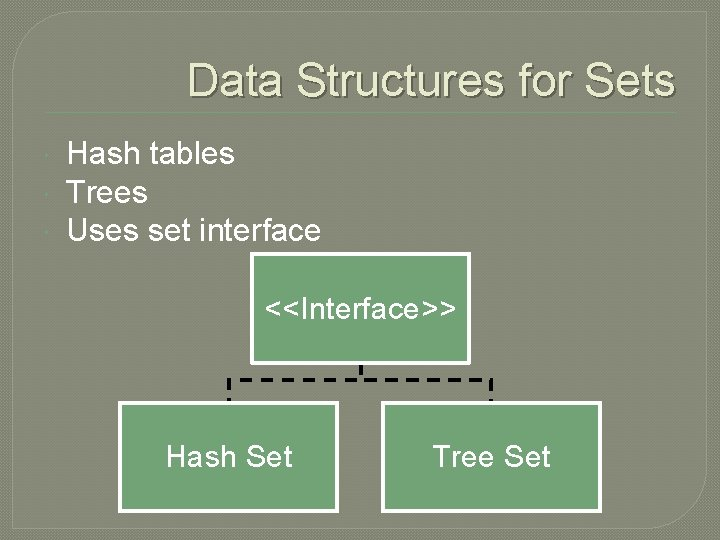Data Structures for Sets Hash tables Trees Uses set interface <<Interface>> Hash Set Tree SetHash Set �Create a Hash Set of Strings Set<String> names = new Hash. Set<String>(); �Adding �Test and deleting names. add(“Romeo”); names. remove(“Juliet”); to see if element is in set if (names. contains(“Juliet”);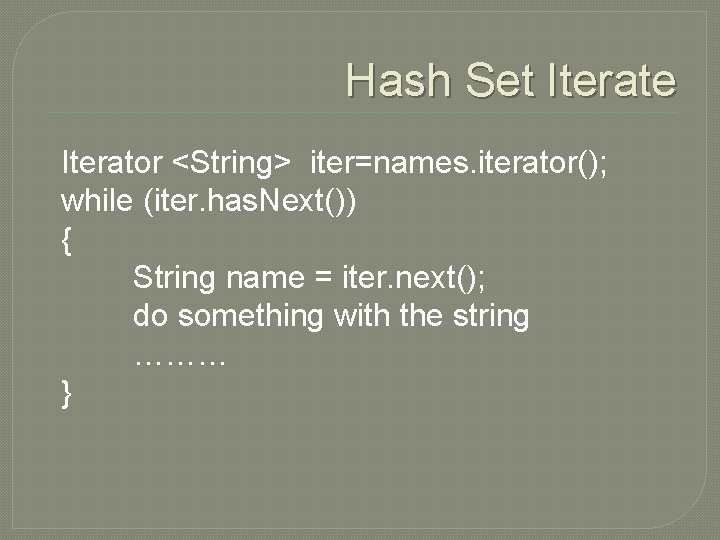Hash Set Iterate Iterator <String> iter=names. iterator(); while (iter. has. Next()) { String name = iter. next(); do something with the string ……… }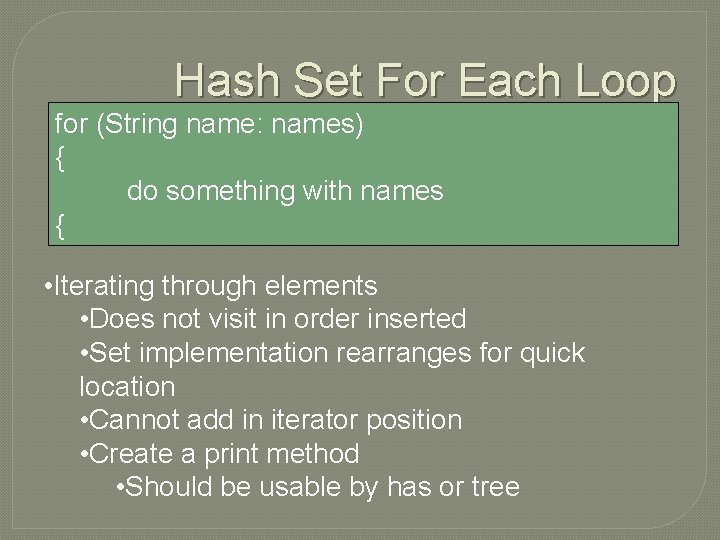Hash Set For Each Loop for (String name: names) { do something with names { • Iterating through elements • Does not visit in order inserted • Set implementation rearranges for quick location • Cannot add in iterator position • Create a print method • Should be usable by has or treeMaps �Data type �Associates keys and values �A function from one set(key) to another set(value) �Every key has unique value �A value may have multiple keys �Two kinds of implementation • Hash Map • Tree Map • Both implement Map Interface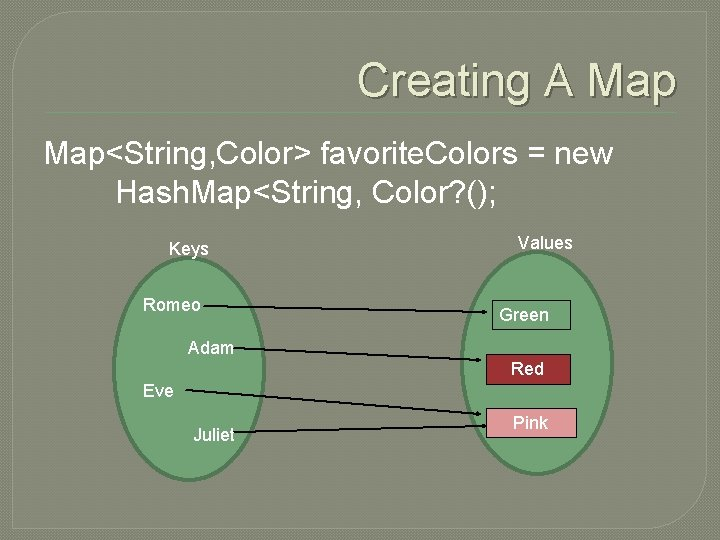Creating A Map<String, Color> favorite. Colors = new Hash. Map<String, Color? (); Keys Romeo Values Green Adam Red Eve Juliet Pink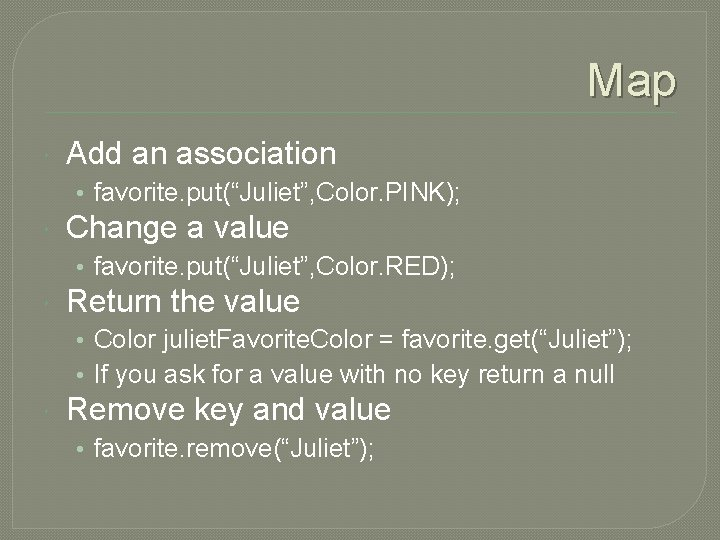Map Add an association • favorite. put(“Juliet”, Color. PINK); Change a value • favorite. put(“Juliet”, Color. RED); Return the value • Color juliet. Favorite. Color = favorite. get(“Juliet”); • If you ask for a value with no key return a null Remove key and value • favorite. remove(“Juliet”);Map Find all keys and values in map Set<String> key. Set = m. key. Set(); For(String key: Key. Set) { Color value=m. get(key); System. out. println(Key + “->” + value); }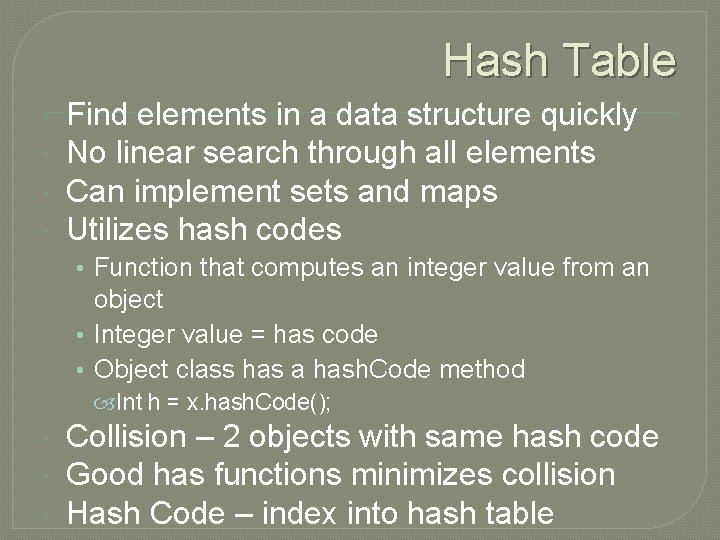Hash Table Find elements in a data structure quickly No linear search through all elements Can implement sets and maps Utilizes hash codes • Function that computes an integer value from an object • Integer value = has code • Object class has a hash. Code method Int h = x. hash. Code(); Collision – 2 objects with same hash code Good has functions minimizes collision Hash Code – index into hash tableHash Table Simplest form • Array index to hash table • In array insert each object @ location of its hash code • If anything already in array – object is present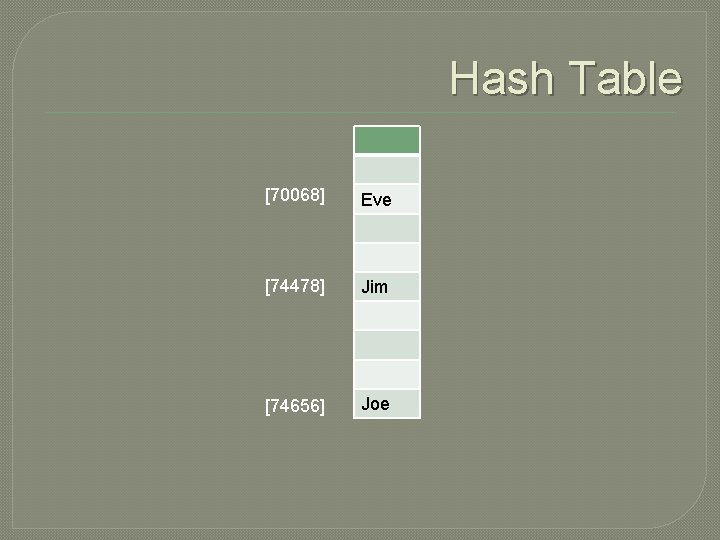Hash Table  Eve  Jim  Joe• Problem with this idea Not possible to allocate an array that is large enough to hold all possible integer index positions Must reduce hash function to fit the array size int h = x. hash. Code(); if (h<0) h = -h; position = h. %buckets. length; This approach increases likely hood of collisions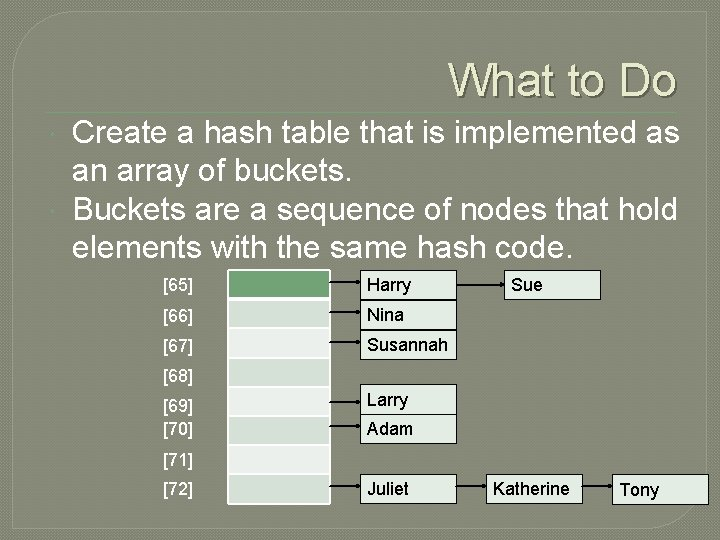What to Do Create a hash table that is implemented as an array of buckets. Buckets are a sequence of nodes that hold elements with the same hash code.  Harry  Nina  Susannah Sue    Larry Adam   Juliet Katherine Tony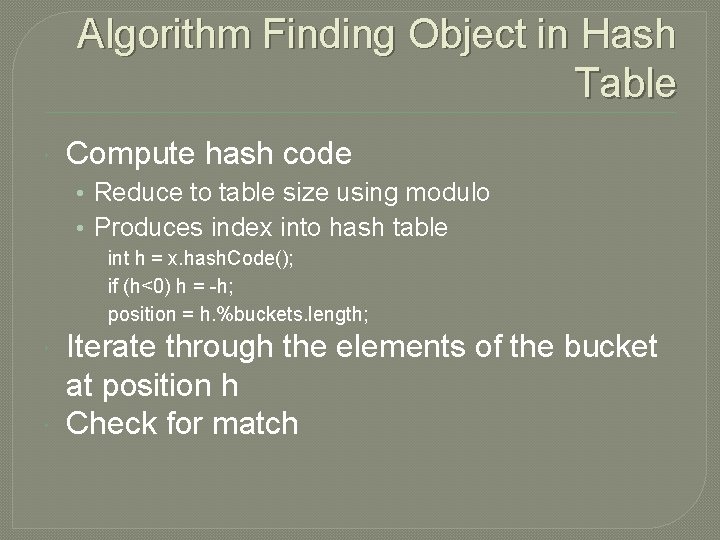Algorithm Finding Object in Hash Table Compute hash code • Reduce to table size using modulo • Produces index into hash table int h = x. hash. Code(); if (h<0) h = -h; position = h. %buckets. length; Iterate through the elements of the bucket at position h Check for matchEfficiency Depends on number of collisions or buckets Few buckets – quick O(1) Many buckets – much slower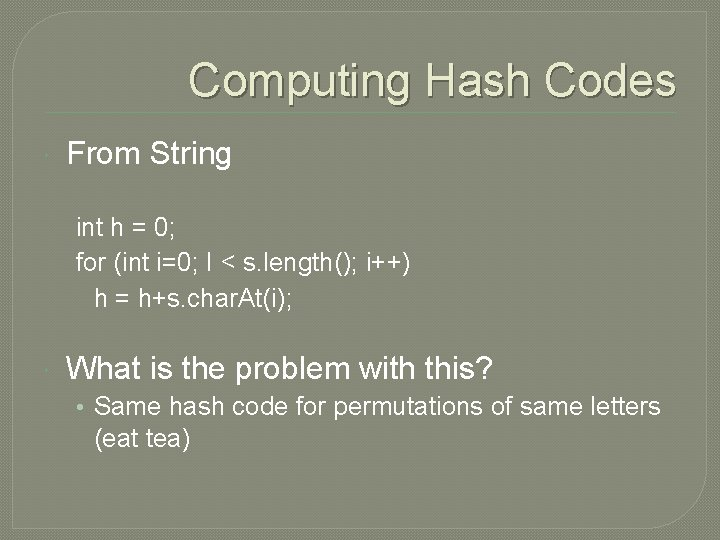Computing Hash Codes From String int h = 0; for (int i=0; I < s. length(); i++) h = h+s. char. At(i); What is the problem with this? • Same hash code for permutations of same letters (eat tea)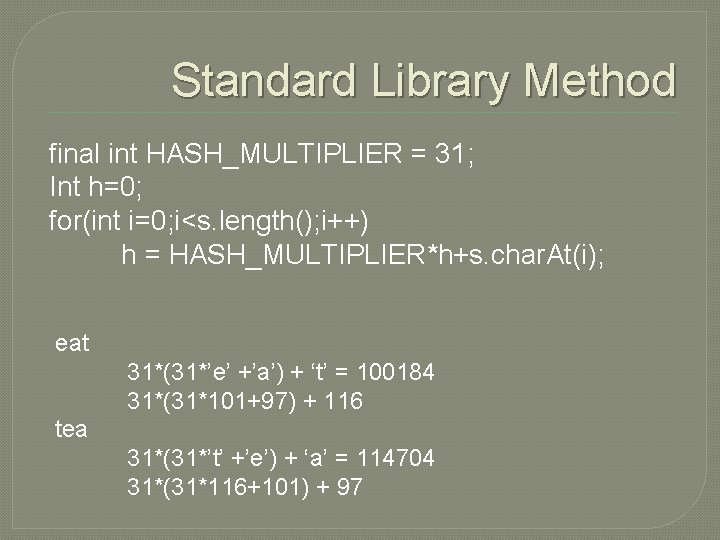Standard Library Method final int HASH_MULTIPLIER = 31; Int h=0; for(int i=0; i<s. length(); i++) h = HASH_MULTIPLIER*h+s. char. At(i); eat 31*(31*’e’ +’a’) + ‘t’ = 100184 31*(31*101+97) + 116 tea 31*(31*’t’ +’e’) + ‘a’ = 114704 31*(31*116+101) + 97How to Create Hash Code for Your Object � Example Coin class public class Coin { final int HASH_MULTIPLIER = 29; public int hash. Code() { int h 1 = name. hash. Code(); int h 2 – new Double(value. hash. Code(); int h = HASH_MULTIPLIER*h 1+h 2; return h; } � In a hash map only the keys are hashed.